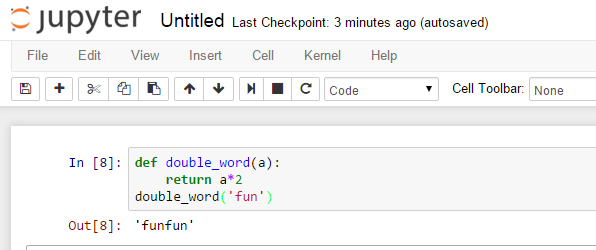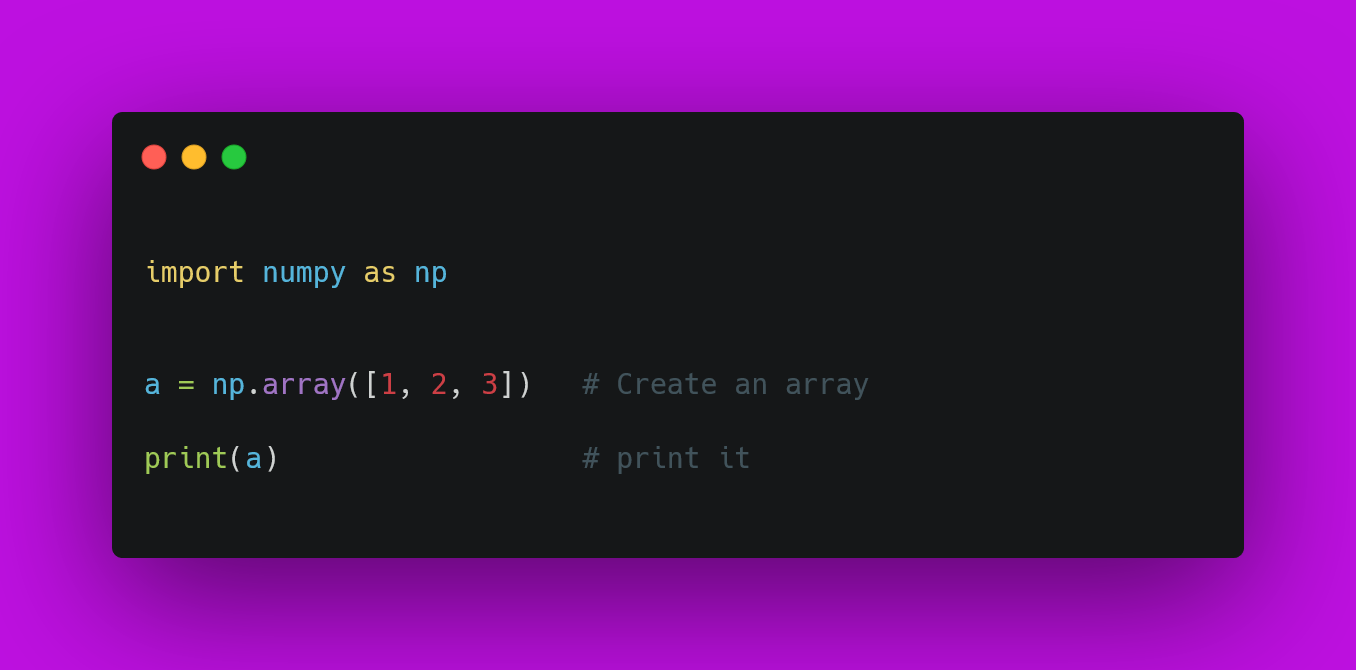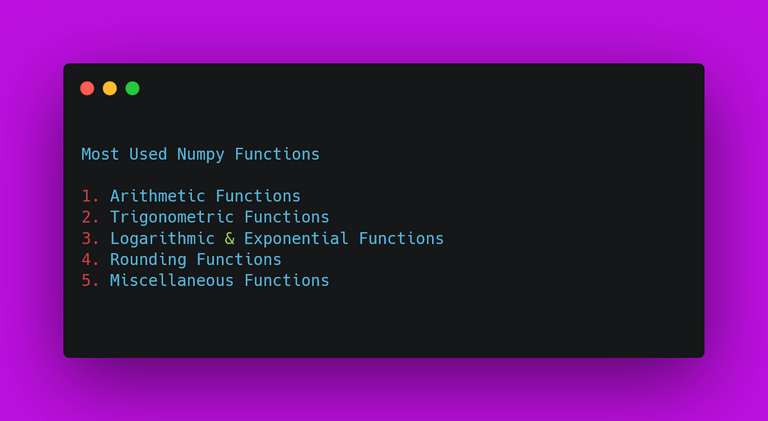in Education6 months ago

In this post, we are going to see How to Use NumPy in Google Colab. Numpy is one of the scientific library that you can use with Python for mathematical and data science computing. Previously in my introductory article on the Google Colab Tutorial, you'd find some interesting things you can learn about the Google Colab notebooks.

I assume that you have gone through my previously pointed article and now have the access to one of the Google Colab notebook. So we are going to take a look at the Numpy library.

There is a lot that you can do and use with the Google Colab. Some of the things that you would be using it for is using it for arrays, dataframes and also for doing the mathematical actions on the same.

I have created a video to give you an overview on how to use the Google Colab and Numpy Library for the data science work. You should give this one a try.

### Open Notebook

By the time you finish the video, you get the idea of what we are going to be doing in this tutorial. And the thing is that we are carrying out everything within the Jupyter notebook. In case of the Google Colab notebook it should look something like the notebook below.### Use Numpy

There is a typical way to install the libraries in the python notebook. In case of the Google Colab that would be different in most of the cases. I'd say in case of the installation you can use the following commands.

!pip install numpy

After you install it and get the message you can go ahead and start testing your code as well. You can type in the below code and see the output by pressing the run button or doing it from the menu.### Numpy functions You Should try Out

Numpy is a vast library and some of the functions you would use a lot. And a lot of those functions you would not want to use. Some of the time that is what leads to solving the problems. I realized that finding use for those functions and using them in your code would save your time for some specific set of the use case.Angle functions, arithmetic functions are the two that you would most commonly used functions. And if you dealing with a lot of float values then you would use the rounding functions. And then there are some of the functions which you would call for the simple math operations which are specific to the logarithmic work too.

Now that you have learned about the Google colab and the Numpy library. You would find some seriously good use for the numpy library with the simple yet complex math operations. Some of the time all that you would have to use is for the simple work that you can quickly do yet stay accurate on the output.

Next I would love to cover the other libraries like matplotlib, pandas and also the pytorch. That would pretty much get you up and moving in the data science work. You can do a lot of data science work from the google colab itself. It saves you money being spent on buying the high end spec computer. As most of the work is done on the cloud.

If you happen to like this content, do give me feedback over there and that would help me improve my efforts in near future.

Sort:#### You have received a 1UP from @gwajnberg!

The @oneup-cartel will soon upvote you with:
@stem-curator, @cent-curator
And they will bring !PIZZA 🍕.